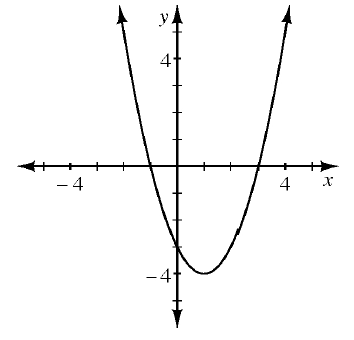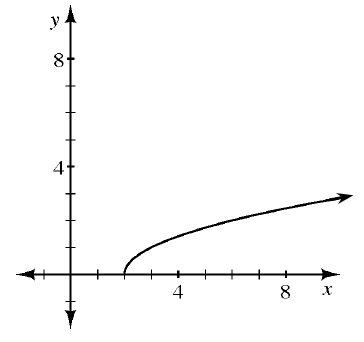### Home > CCAA8 > Chapter 8 Unit 9 > Lesson CCA: 8.1.2 > Problem8-18

8-18.

For each rule represented below, state the $x$- and $y$-intercepts, if possible.

1.The $x$-intercept is where $y = 0$.
The $y$-intercept is where $x = 0$.

1.1.  $x$ $y$ $-5$ $-4$ $-3$ $-2$ $-1$ $0$ $1$ $2$ $8$ $4$ $0$ $-4$ $0$ $2$ $0$ $-4$

Look for the zeros in the table.

1. $5x-2y=40$

The $y$-intercept is $(0, y)$ and the $x$-intercept is $(x, 0)$.